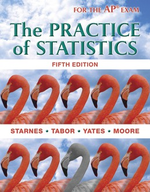×
Get Full Access to The Practice Of Statistics - 5 Edition - Chapter 7.3 - Problem 53
Get Full Access to The Practice Of Statistics - 5 Edition - Chapter 7.3 - Problem 53

×

# Larger sample Suppose that the blood cholesterol level of all men aged 20 to 34 followsISBN: 9781464108730 488

## Solution for problem 53 Chapter 7.3

The Practice of Statistics | 5th Edition

• Textbook Solutions
• 2901 Step-by-step solutions solved by professors and subject experts
• Get 24/7 help from StudySoup virtual teaching assistantsThe Practice of Statistics | 5th Edition

4 5 1 391 Reviews
13
0
Problem 53

Larger sample Suppose that the blood cholesterol level of all men aged 20 to 34 follows the Normal distribution with mean m = 188 milligrams per deciliter (mg/dl) and standard deviation s = 41 mg/dl. (a) Choose an SRS of 100 men from this population. Describe the sampling distribution of x . (b) Find the probability that x estimates m within 3 mg/dl. (This is the probability that x takes a value between 185 and 191 mg/dl.) Show your work. (c) Choose an SRS of 1000 men from this population. Now what is the probability that x falls within 3 mg/dl of m? Show your work. In what sense is the larger sample better?

Step-by-Step Solution:

Step 1 of 4

Define a random variable for blood cholesterol level in men aged 20 to 34.

Then,Step 2 of 4

Step 3 of 4

##### ISBN: 9781464108730

This full solution covers the following key subjects: . This expansive textbook survival guide covers 44 chapters, and 1409 solutions. The answer to “Larger sample Suppose that the blood cholesterol level of all men aged 20 to 34 follows the Normal distribution with mean m = 188 milligrams per deciliter (mg/dl) and standard deviation s = 41 mg/dl. (a) Choose an SRS of 100 men from this population. Describe the sampling distribution of x . (b) Find the probability that x estimates m within 3 mg/dl. (This is the probability that x takes a value between 185 and 191 mg/dl.) Show your work. (c) Choose an SRS of 1000 men from this population. Now what is the probability that x falls within 3 mg/dl of m? Show your work. In what sense is the larger sample better?” is broken down into a number of easy to follow steps, and 114 words. This textbook survival guide was created for the textbook: The Practice of Statistics, edition: 5. The full step-by-step solution to problem: 53 from chapter: 7.3 was answered by , our top Statistics solution expert on 03/19/18, 03:52PM. Since the solution to 53 from 7.3 chapter was answered, more than 338 students have viewed the full step-by-step answer. The Practice of Statistics was written by and is associated to the ISBN: 9781464108730.

## Discover and learn what students are asking

Unlock Textbook Solution

Enter your email below to unlock your verified solution to:

Larger sample Suppose that the blood cholesterol level of all men aged 20 to 34 follows